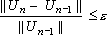﻿ Convergence Criteria
Manufacturing Solutions

# Convergence Criteria# Convergence CriteriaThe governing equations of HX-Forge are nonlinear due to the fact that viscosity is dependent on the temperature and shear rate.

The presence of these nonlinearities requires the use of some iterative technique to solve the problem. In HX-Forge, in each iteration, nonlinear terms are evaluated using the solution from the previous iteration. Hence, the system becomes linear and can be solved after imposing boundary conditions. This procedure, called Picard method, is continued until the difference between two successive iterative solutions is less than a specified value (ex: 0.001). In other words, the solution is said to have converged if the ratio of the norm of the change in solution between two successive nonlinear iterations (ex: (n-1) and n) to the norm of the solution at the previous nonlinear iteration (n-1) falls below a prescribed small value. Mathematically:where, ε is the user specified tolerance. ε = 0.001 implies that the change in the solution between two successive time steps is 0.1 percent . When solving the problem using pressure-splitting scheme, this norm is computed after every time step. After each nonlinear iteration/time step, HX-Forge computes magnitude (norm) for each variable (velocity vector, pressure, and temperature). These values indicate the influence of each quantity on the error norm calculations and overall convergence behavior. A sample output showing the convergence behavior is shown here.

### Norm of Change in Solution

(S)

||S[n]||

||S[n]|| - ||S[n-1]||

||S[n-1]||

||S[n] - S[n-1]||

||S[n-1]||

Velocity

5.991262e-05

-1.617996e-03

4.018664e-03

Pressure

9.656620e-06

3.165889e-04

1.129513e-02

Temperature

8.894094e+01

-2.645006e-08

1.165693e-06

Total

8.894094e+01

-2.645006e-08

1.165700e-06

In this table, Change in Solution Norm indicates the percentage change in the magnitude of the solution over two successive time steps. The Solution Norm and Change in Solution Norm quantities are computed to provide additional insight into the convergence behavior. The Total Norm of Change in Solution includes all components, and is the most stringent of all the norms computed by the solver. The solver checks for this norm to determine the convergence.

The parameter MaxNonlinearIters defines the maximum number of nonlinear iterations. In the case of steady state simulations, the program stops whenever the convergence criterion is satisfied before completing the MaxNonlinearIters. Otherwise, HX-Forge completes the MaxNonlinearIters and prints a statement to this effect. The same is true for unsteady flow simulations before the calculations are advanced to the next time step. The default value for this parameter is 25.

The parameter NonlinIterTolerance specifies the acceptable convergence criterion for nonlinear iterations. The default value for this parameter is set to 0.001 and it indicates that the acceptable error in the solution norm is 0.1 percent . To accelerate the convergence of the Picard iteration technique, HX-Forge uses a weighted average of the solutions from last two iterations to evaluate the nonlinear coefficients:

Un = βUn+ (1 - β) U(n-1)

where, β is the relaxation/weighting factor and 0 < β < 1. The parameters RelaxComp1, RelaxComp2, and RelaxComp3 are used to define the relaxation/weighting factors for the velocities, pressure, and temperature, respectively.